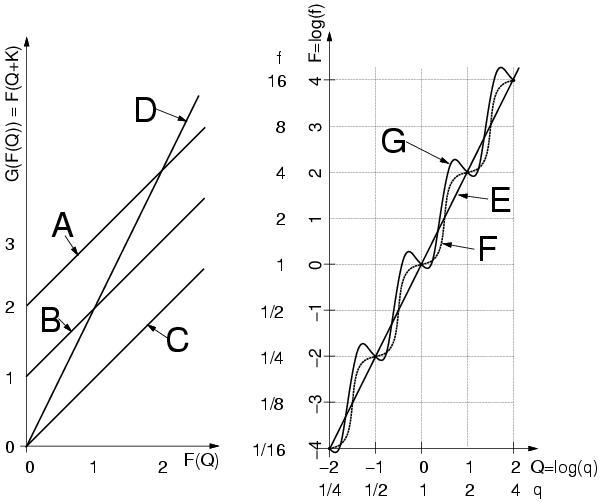Department of Electrical and Computer Engineering
University of Toronto

ECE 431 (ECE431H1F), Digital Signal Processing (DSP); Prof. S. Mann

Thursday December 13, 2001, 9:30am, in room HA-410

Type X (aids allowed''); there are five questions worth equal weight.

Note: Please write your answers in the answer book only. All answers should be properly explained by providing appropriate detailed arguments, reasoning, formulas, diagrams, etc..

Q1a Let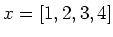; calculate the 4 point discrete Fourier transform, X=fft(x). Compute the real part,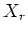of X, the imaginary part,of X, the even part,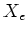of X, and the odd part,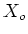of X. Hint: each of these four answers should contain four samples.

Q1b Compute the 2 point DFT of the following signals: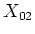being the DFT of [1, 3] andbeing the DFT of [2, 4].

Q1c Is the 2 point DFT of a real signal of length 2 always real, and if so, why?

Q1d Show howcan be reconstructed using the results in Q1b. Hint: write each sample ofas a linear combination of the samples ofand.

Q1e Now consider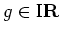, where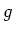is an odd function. (Recall that for an odd function,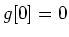and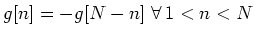, whereis the number of samples in.) Ifhas a length of four samples, how many degrees of freedom are there in?

Q2a Consider a real signal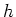having an arbitrary length. We desire to find thepoint DFT of, as defined by: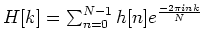, using a single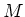point DFT. What's the minimumrequired, given no additional knowledge of.

Q2b Show how you can compute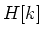using a single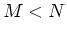point DFT. Hint: consider a decimation-in-time or decimiation-in-frequency approach, combined with some thoughts on question 1.

Q2c In situations whereis an odd function, what is the minimumrequired to obtain theDFT offrom thepoint DFT of.

Q2d Show how you would obtain thepoint DFT of a real and odd function using a singlepoint DFT, whereis as found in Q2c.

Q3 Design a single pole causal lowpass filter,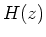having real coefficients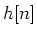and having -40dB gain at 22.05kHz. You are to use the bilinear transform, with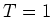. For your final design, provide bothand its impulse response.

Q4a Explain how you would rescale an array having dimension 400 (down) by 600 (across) up to an array having dimensions 480 by 640. Hint: recall the resampling (resizing) done in lab 2.

Q4b Suppose that you measure the same array quantity again and again in a large number of separate measurement trials. The measurements are quite similar but slightly different due to random noise. Would it be best to simply select one of the measurement arrays at random, or is there a better approach than just selecting one at random. Explain your reasoning. Hint: recall lab 1.

Q5a Compute the comparagram of the following two image arrays:

image 0      image 1

1 3 2 3      2 3 3 3
1 2 1 2      1 2 2 2
0 0 2 0      0 1 3 1


Q5b With reference to the figure below, which of the following comparametric plots at left correspond to possible candidate solutions shown at right. Identify which solutions correspond to which comparametric plots, if any, for any that are solutions. Explain your reasoning. Note that logarithms are base 2, and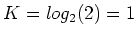.